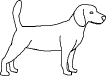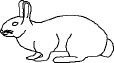EnchantedLearning.com is a user-supported site.
As a bonus, site members have access to a banner-ad-free version of the site, with print-friendly pages.

 Go to the AnswersMath Pages EnchantedLearning.com Divide Ten Pets Divide the pets into groups to do division. Visual Division PagesDivision Pages

 Divide the dogs into two equal groups.How many dogs are there? How many groups of dogs are there? How many dogs are in each group?
10 ÷ 2 =

 Divide the cats into five equal groups.How many cats are there? How many groups of cats are there? How many cats are in each group?
10 ÷ 5 =

 Divide the rabbits into ten equal groups.How many rabbits are there? How many groups of rabbits are there? How many rabbits are in each group?
10 ÷ 10 =

## Enchanted Learning Search

 Search the Enchanted Learning website for: